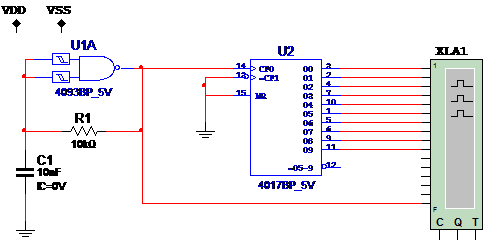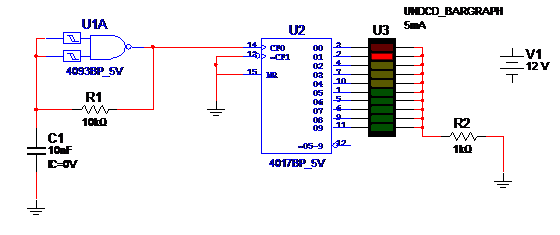### DatasheetsN° of component

## Divide-by-10 Using the 4017 (NI001E)

In this simulation we use one gate of a 4093 as a low frequency oscillator producing square pulses in its output. The pulses are applied to a 4017 connected as a divide-by-10 circuit. The pulses in the outputs of the 4017 are applied to the Logic Analyzer of the NI Multisim.

The 4017 has 10 outputs, one each time going to the high logic level commanded by the pulses of the 4093. The output can be used to drive LEDs or power stages in a sequencer, for instance.The frequency is given by the capacitor C1. Change to have best resulting the simulation. R1 also can be changed in the range between 10 k ohm and 1 M ohm. Figure bellow shows how to replace the logic analyzer by a LED bargraph.